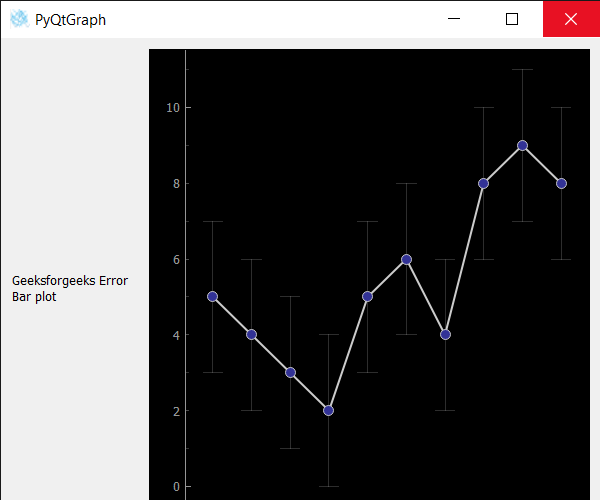# PyQtGraph – Setting Opacity Property of Error Bar Graph

• Last Updated : 22 Nov, 2021

In this article we will see how we can set opacity property of the error bar graph in the PyQtGraph module. PyQtGraph is a graphics and user interface library for Python that provides functionality commonly required in designing and science applications. Its primary goals are to provide fast, interactive graphics for displaying data (plots, video, etc.). Error bars are graphical representations of the variability of data and used on graphs to indicate the error or uncertainty in a reported measurement. They give a general idea of how precise a measurement is, or conversely, how far from the reported value the true value might be. Opacity is the measure of impenetrability to electromagnetic or other kinds of radiation, especially visible light. In radiative transfer, it describes the absorption and scattering of radiation in a medium, such as a plasma, dielectric, shielding material, glass, etc.
We can create a plot window and create error bar graph on it with the help of commands given below

```# creating a pyqtgraph plot window
plt = pg.plot()

# creating a error bar item object
error = pg.ErrorBarItem(x=x, y=y, top=top, bottom=bottom, beam=0.5)```

In order to do this we use setOpacity method with the error bar item object
Syntax : error.setOpacity(n)
Argument : It takes float value as argument
Return : It returns None

Attention geek! Strengthen your foundations with the Python Programming Foundation Course and learn the basics.

To begin with, your interview preparations Enhance your Data Structures concepts with the Python DS Course. And to begin with your Machine Learning Journey, join the Machine Learning - Basic Level Course

Below is the implementation

## Python3

 `# importing Qt widgets``from` `PyQt5.QtWidgets ``import` `*` `# importing system``import` `sys` `# importing numpy as np``import` `numpy as np` `# importing pyqtgraph as pg``import` `pyqtgraph as pg``from` `PyQt5.QtGui ``import` `*``from` `PyQt5.QtCore ``import` `*` `from` `collections ``import` `namedtuple` `class` `Window(QMainWindow):` `    ``def` `__init__(``self``):``        ``super``().__init__()` `        ``# setting title``        ``self``.setWindowTitle(``"PyQtGraph"``)` `        ``# setting geometry``        ``self``.setGeometry(``100``, ``100``, ``600``, ``500``)` `        ``# icon``        ``icon ``=` `QIcon(``"skin.png"``)` `        ``# setting icon to the window``        ``self``.setWindowIcon(icon)` `        ``# calling method``        ``self``.UiComponents()` `        ``# showing all the widgets``        ``self``.show()` `    ``# method for components``    ``def` `UiComponents(``self``):` `        ``# creating a widget object``        ``widget ``=` `QWidget()` `        ``# creating a label``        ``label ``=` `QLabel(``"Geeksforgeeks Error Bar plot"``)` `        ``# setting minimum width``        ``label.setMinimumWidth(``130``)` `        ``# making label do word wrap``        ``label.setWordWrap(``True``)` `        ``# setting configuration options``        ``pg.setConfigOptions(antialias``=``True``)` `        ``# creating x-axis values``        ``x ``=` `np.array([``1``, ``2``, ``3``, ``4``, ``5``, ``6``, ``7``, ``8``, ``9``, ``10``])` `        ``# creating y-axis values``        ``y ``=` `np.array([``5``, ``4``, ``3``, ``2``, ``5``, ``6``, ``4``, ``8``, ``9``, ``8``])` `        ``# creating upper bound values``        ``top ``=` `np.array([``2``, ``2``, ``2``, ``2``, ``2``, ``2``, ``2``, ``2``, ``2``, ``2``])` `        ``# creating lower bound values``        ``bottom ``=` `np.array([``2``, ``2``, ``2``, ``2``, ``2``, ``2``, ``2``, ``2``, ``2``, ``2``])` `        ``# creating a plot window``        ``plt ``=` `pg.plot()` `        ``# creating a error bar item``        ``error ``=` `pg.ErrorBarItem(beam``=``0.5``)` `        ``# setting data to error bar item``        ``error.setData(x``=``x, y``=``y, top``=``top, bottom``=``bottom)` `        ``# adding error bar item to the plot window``        ``plt.addItem(error)` `        ``# plotting the data on plot window``        ``plt.plot(x, y, symbol``=``'o'``, pen``=``{``'color'``: ``0.8``, ``'width'``: ``2``})` `        ``# Creating a grid layout``        ``layout ``=` `QGridLayout()` `        ``# minimum width value of the label``        ``label.setMinimumWidth(``130``)` `        ``# setting this layout to the widget``        ``widget.setLayout(layout)` `        ``# adding label in the layout``        ``layout.addWidget(label, ``1``, ``0``)` `        ``# plot window goes on right side, spanning 3 rows``        ``layout.addWidget(plt, ``0``, ``1``, ``3``, ``1``)` `        ``# setting this widget as central widget of the main window``        ``self``.setCentralWidget(widget)` `        ``# setting opacity of error bar item``        ``error.setOpacity(``0.3``)` `# create pyqt5 app``App ``=` `QApplication(sys.argv)` `# create the instance of our Window``window ``=` `Window()` `# start the app``sys.exit(App.``exec``())`

Output :My Personal Notes arrow_drop_up